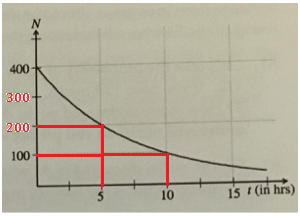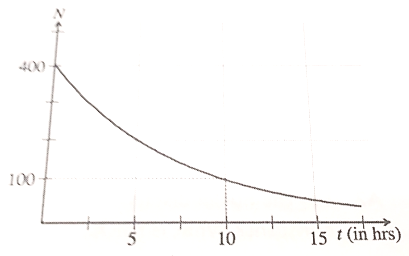# Problem: The curve to the right shows the radioactive decay of a particular sample of a nucleus called Element A. A particular nucleus survives for the first five hours, what's the probability that particular nucleus of Element A will decal between hours 5 and 10? What is the probability that particular nucleus of element A will decay between 5 and 20 hours?

###### FREE Expert Solution

We have to determine the probability of decay of a certain element A between certain time intervals using the given graph.

Radioactive decay is a process whereby an unstable nucleus undergoes breakdown to form relatively stable nuclei.

The time that it takes for half of a radioactive substance to decay is called the half-life.

We will start by labelling the given graph so that it is easier to read and extract values from it.95% (222 ratings)###### Problem Details

The curve to the right shows the radioactive decay of a particular sample of a nucleus called Element A. A particular nucleus survives for the first five hours, what's the probability that particular nucleus of Element A will decal between hours 5 and 10? What is the probability that particular nucleus of element A will decay between 5 and 20 hours?